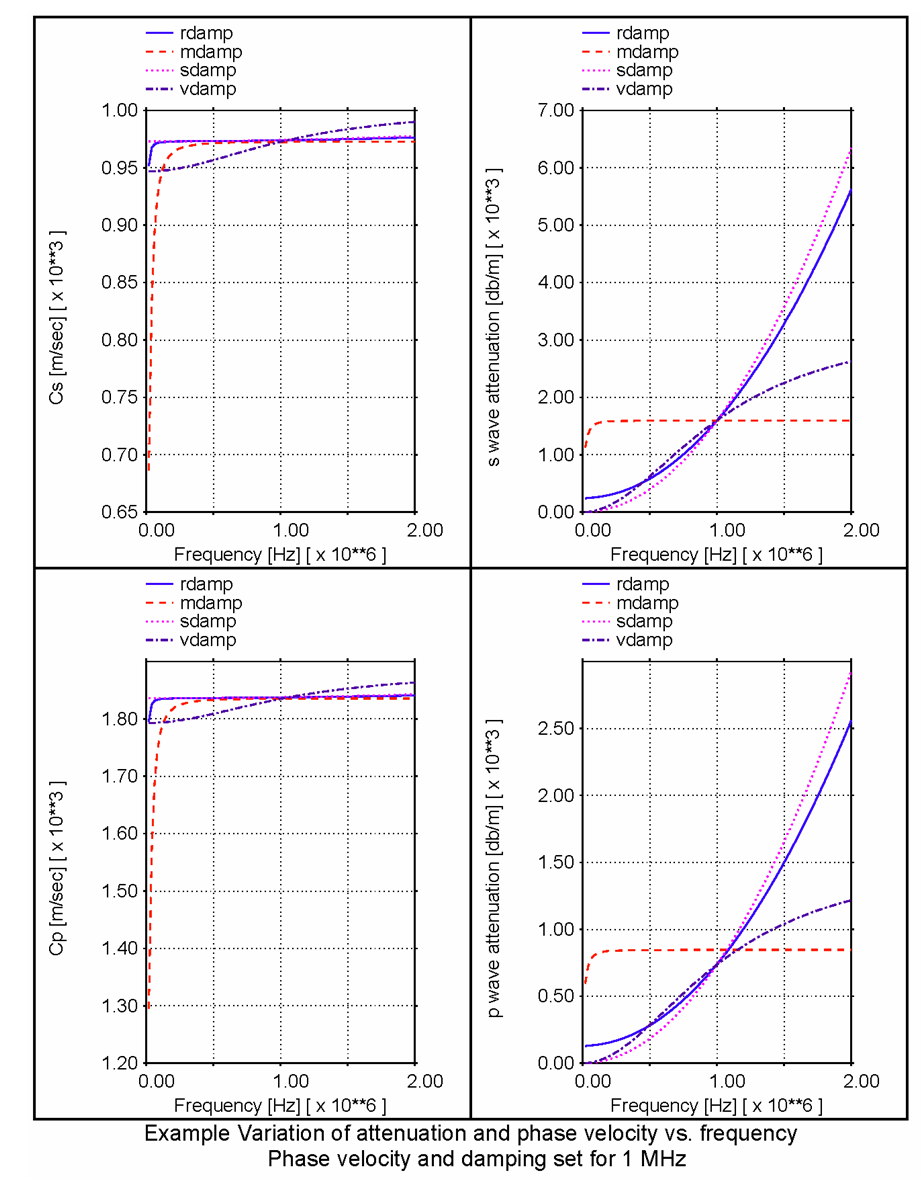# What is Material Damping?

## Background

Experiments show that many real materials are lossy, i.e. they convert some mechanical energy to heat as they deform. Regardless of the actual micro scale physical process, it is convenient to represent this energy conversion via viscous damping. Here we highlight some of the issues which arise in selecting an appropriate viscous damping model.

Frequency dependence - The amount of damping observed in a particular material typically varies with frequency. One should choose a damping model which reasonably approximates the observed damping over the frequency range of interest. Phase velocity may also vary with frequency. If this effect is significant, the elastic moduli should be adjusted to best approximate the phase velocity over the frequency range of interest.

Shear / volumetric issues - The observed damping could be due to either shear distortions, volume changes or both. Typically, shear distortions are the primary source of energy dissipation. Note that a shear wave induces only shear distortions, but a longitudinal wave induces both shear and volumetric deformations. In fact, much of the damping observed in a longitudnal wave is frequently due to shear distortions.

Stability - Damping increases the impedance of a material, thus reducing its stable timestep. Among the models discussed below, the nwtnsdmp and rdmp models require a smaller timestep than the vdmp model, whereas the mdmp model permits the largest timestep.

## Damping Measures

A number of damping measures are commonly used, and unfortunately this sometimes causes confusion. The important distinctions are whether the measure describes wave amplitude or energy, and whether the loss is per cycle or per unit distance. Here we list some common damping measures, and how they relate.

### Attenuation Coefficient (α):

As a plane wave of angular frequency ω propagates through a lossy material, it’s amplitude (stress or velocity) decays exponentially with distance, e.g.: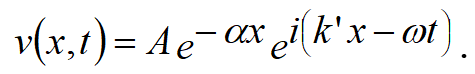Some authors use α to denote energy loss per unit distance.

• k' is termed the wave number.
• At any given frequency, it is related to the phase velocity: c = ω / k'.
• We will use a complex wave number: k = k' + iα
• In our notation, the energy varies as e-2 αx
• Both the damping and phase velocity vary with frequency, so we describe materials in terms of their elastic constants: shear modulus (G), bulk modulus (K) and constrained modulus (B= K+4/3G).

### dB loss:

The dB is a measure of power loss. If A1 is the incident plane wave amplitude and A2 is the amplitude after it propagates a unit distance, then the dB loss per unit distance is related to the attenuation coefficient: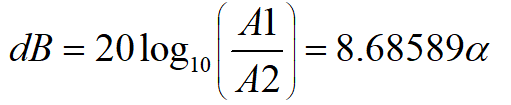### Quality Factor (Q):

The quality factor (Q) is a measure of the rate of power absorption. For small α: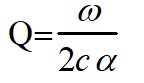where c denotes the phase velocity

### Log Decrement (δ):

The log decrement δ represents the amplitude decay per cycle: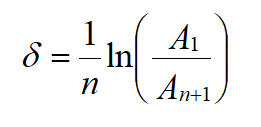### Fraction of Critical Damping (D):

Critical damping is a concept that evolved from the study of a simple harmonic oscillator. It is the damping value at which the solution changes from monotonically decaying (no oscillations) to damped oscillations. Thus for D < 1, damped oscillations will be observed, whereas for D = > 1 the solution will decay monotonically. By analogy with the simple harmonic oscillator, we define the fraction of critical damping as: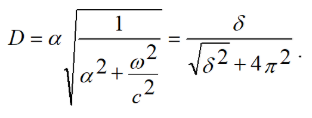## Damping Models in OnScale

### Mass Proportional Damping (MDMP):

This damping operates on the nodal velocities directly rather than on the strain rates. It may be envisioned as connecting each node to a stationary datum with independent dashpots. It is characterized by a single constant (γ). Given, (G, K, ρ); γ would typically be chosen by matching the damping of one wave type at a selected frequency. Because it operates on the velocities rather than the strain rates, the deviatoric and volumetric damping are not independent.

The complex wave number for a p-wave is: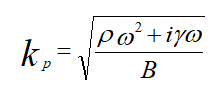From this, the attenuation coefficient is: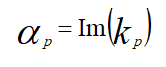The phase velocity is: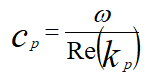Likewise for a shear wave: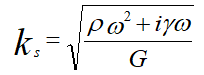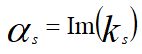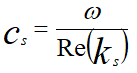Here ρ is the density and γ is the damping constant and B is the constrained modulus (= K+4/3G). mdmp sets the coefficient γ to match the requested damping for the specified wave type at the requested frequency.

### Newtonian Viscosity (NWTN):

Shear and volumetric Newtonian viscosities are specified for a material via the matr nwtn subcommand. In addition to the stresses due to strains, this model adds stresses which are linearly proportional to the strain rates.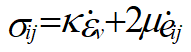Here, κ and μ are the bulk and shear viscosity coefficients respectivel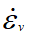and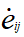are the volumetric and deviatoric strain rates. Though the stiffness may be anisotropic, the viscosities are currently assumed isotropic. This is the continuum analog of the Kelvin (or Voigt) model.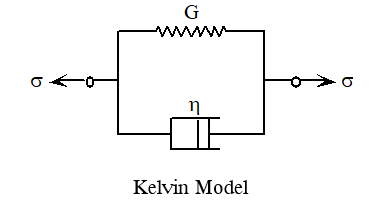This model produces a dB loss per unit distance that increases as the square of the frequency for small to moderate damping values: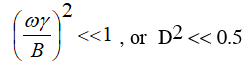Above this level, the rate of increase begins to level off. The variation of phase velocity with frequency is negligible for small to moderate damping values.

The complex wave number for a p-wave is: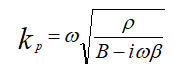From this, the attenuation coefficient is: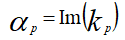The phase velocity is: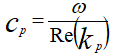Likewise for a shear wave: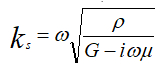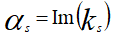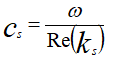Here, B (=K+4/3G) is the constrained modulus, β = κ + 4/3μ is the constrained viscosity, ρ is the density and ω is the angular frequency. For large damping values, this model requires a very small timestep compared to an undamped material with the same elastic moduli. Typically, μ is selected to match the shear damping at a given frequency, then β is set to match the longitudinal wave.

### Stiffness Proportional Damping (SDMP):

This option is identical to Newtonian Viscosity, except that a more convenient method of specifying the viscosities has been provided. Stiffness-Proportional damping is specified for a material via the matr sdmp subcommand. sdmp adjusts the viscosity and stiffness parameters to match the requested wavespeed and attenuation for both wave types at the requested frequency.

### Rayleigh Damping (RDMP):

rdmp combines mdmp and sdmp. In addition to matching the wavespeeds and attenuations at the requested frequency, OnScale also tries to minimized a weighted error in attenuation over a frequency range specified on the frng command.

The complex wave number for a p-wave is: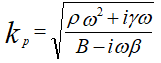From this, the attenuation coefficient is: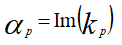The phase velocity is: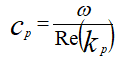Here, B =K+4/3G is the constrained modulus, β = κ + 4/3μ is the constrained viscosity.

Likewise for a shear wave: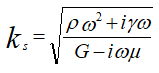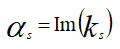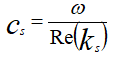### Viscous Damping (VDMP):

This model implements the continuum analog of the standard linear solid.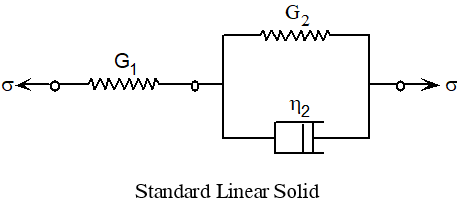The deviatoric stress and pressure rates due to the damping model are: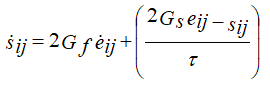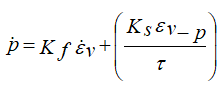Here, Gf and Kf are the moduli for high rate loading, Gs and Ks are the moduli for low rate loading, and the τ is the relaxation time. With respect to the sketch, the high rate loading modulus corresponds to the G1 spring. The low rate modulus corresponds the series combination of G1 and G2: Gs = 1/ ( 1/G1 + 1/G2). The relaxation time is related to the lumped parameter elements as τ = η / (G1+G2) As with the previous model, shear and/or volumetric damping may be specified. This model produces a dB loss per unit distance which is approximately linear over a wide frequency range.

At large damping values, the phase velocity variation with frequency can also be noticeable. As with sdmp, the code attempts to pick coefficients that match the requested wavespeeds and attenuations at the specified frequency.

For a shear wave: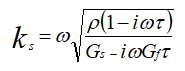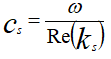Likewise for a longitudinal wave: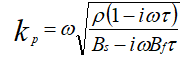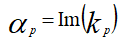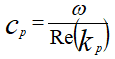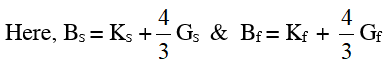### Damping Model - Performance Graphs:

The following figure illustrates the variation of phase velocity and attenuation versus frequency when set to match at 1 Mhz.